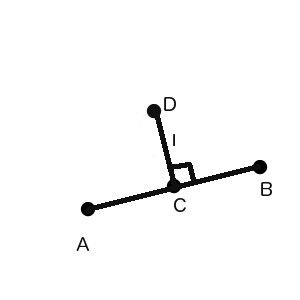# Calculate point coords which lays on normal vector

Hello. I have A and B points which z coord the same. I need calculate point C coords between this two points and calculate D point coords, which lays on normal vector to AB vector, and on length l.I can calculate C point coords using
A.transform.position + (B.transform.position - A.transform.position) / 2f
but how can I do other stuff (calculate D point coords)? (this is 2d game and z coords always 0 for all points)
thanks.

If you get a cross between (B-A) and whatever is the ‘up’ vector for your points (the vector pointing to you from your screen), then this will give you a vector (which we will call ‘N’) that points perpendicular to the line A->B. If you normalize ‘N’, then

`D = C + N * distance to D`

As @Prodigga indicates, the cross product is a good way to go to solve this problem. Given you have positions A and position C (which you calculate in your question) here is a bit of code:

``````var v3AC = v3A - v3C;
var v3D  = v3C + (Vector3.Cross(v3AC, Vector3.forward)).normalized;
``````

You can change which side of the line the point is on by either changing the order of the parameters in the Vector3.Cross() or by using Vector3.back instead of Vector3.forward.